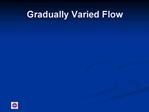DownloadDownload PresentationTélécharger la présentation- - - - - - - - - - - - - - - - - - - - - - - - - - - E N D - - - - - - - - - - - - - - - - - - - - - - - - - - -
Presentation Transcript

1. Gradually Varied Flow Steady flow � no change with time Flow varies slowly along a channel Used to describe flow profiles upstream or downstream of structures, obstructions etc. Bed slope and friction are important

2. Derivation of Equations

3. Assumptions Streamlines have negligible curvature, i.e. no noticeable convergence or divergence, and so the pressure distribution remains hydrostatic; Velocity is uniformly distributed over the channel cross section; The slope of the energy line may be found from uniform flow formulae such as Manning, using the velocity and the hydraulic mean depth which exist at the section under consideration ( or the slope of the energy line at a particular depth at a particular section, is the same as the slope of the bed which would sustain uniform flow at that depth); The slope of the channel is so small that any difference between depths measured vertically and perpendicular to the channel bed may be neglected.

4. GVF is still steady flow so Total head at section 1: (no cos ? and no a � assumptions 4 and 2 above) Differentiating along channel:

5. Now and so Also so and Hence

6. Back substituting: and and So:

7. GVF Equation Equation is for change in surface level along channel. S0 = bed slope, Sf = friction slope. K0 is the conveyance you would have at normal depth in the channel, determined from S0; K is the conveyance you have at the actual observed depth at any point determined from Manning knowing Q, y and B.

8. Notes There are many solutions, some analytical (for prismatic channels) Usually solve numerically � Direct Step or Standard Step methods Application software such as HEC-RAS You need a known depth to start from. This is typically a structure such as a weir

9. Direct Step Method Start at known level Accuracy depends on size of step ?y

10. Direct Step Example A trapezoidal channel has a bed width of 7m, bed slope = 0.0247, side slopes 1:2 (vert.:horiz.), Manning's n=0.03 and carries a flow of 12 cumecs. The water depth behind an underflow sluice placed across the channel is 1.2m. Find the maximum distance upstream from the sluice where irrigation water can be drawn off if this requires at least 0.8m water depth in the channel.

11. Standard Step Method Again, start from known depth Compute ?y at defined intervals ?x Iterative solution, as both E and Sf are unknown at x + ?x

12. Standard Step Example Repeat previous example Use a spreadsheet solution

13. Standard Step Complexities Can deal with natural channels � A, P etc can vary between each station Can deal with flood plains � though have to check with each iteration if you are in the flood plain or the main channel only Often implemented in commercial software

14. Reference Chadwick and Morfett Derivation pages 154-155 Direct Step Method 160-166 Standard Step Method 160-169

15. HEC-RAS River Analysis System

16. Web Link http://www.hec.usace.army.mil/ 2 Useful programmes HEC-RAS River Analysis System Also HEC-HMS Hydrologic Modelling System

17. Brief Demonstration of HEC-RAS Tweed Study Meaning of cross-section data

19. More on Solving the GVF equation Sometimes work downstream and sometimes up depending on known boundary condition. In each case the other direction won�t work Need to classify flow to start solving the problem

21. Types of Profile in Prismatic Channels Direction and relative magnitude of bed slope Actual depth relative to normal depth and critical depth

22. Sustaining or positive slope (S) Mild � subcritical flow (at given Q) Critical � critical flow Steep � supercritical flow Horizontal slope (H) Adverse slope (A)

23. Three depth classifications: Region 1 y > (yo & ycr) Region 2 y lies between yo & ycr �Region 3 y < (yo & ycr)

25. Key Points on Flow Classification and GVF Flow will always try to revert to Normal Depth y0 If in moving towards normal depth, the flow passes through critical depth, a hydraulic jump or hydraulic drop will occur Essential first steps are to calculate the critical depth and normal depth for each reach of channel

26. Summary You need to know what to expect before applying equations, or you might get it wrong!

27. Reference Chadwick and Morfett pages 149-159 Software � HEC-RAS US Army Corps of Engineers http://www.hec.usace.army.mil/

28. Channel with Free Inflow Inflow dependent on head differential Head differential dependent on flow in channel

29. Neglecting friction and change in bed level from 1 to 2: Hence: Also for a rectangular channel: So: But if it is a free outfall and there is normal depth in the channel: Thus we have: Which can be solved for y2

30. Inflow Plot of y2 against q for fixed y1

31. Flow in Channel Plot of y2 against q

32. Combination Superimpose the two plots for fixed y1. Max q at critical depth in the channel.

33. Example A large reservoir discharges into a wide rectangular channel that has a bed slope of 0.0005, and a Manning�s �n� of 0.025. The reservoir level is 100 maod, and the elevation of the bed of the channel at the reservoir is 98.0 maod. Determine the free discharge per unit width from the reservoir. �

34. Reference None for this section.